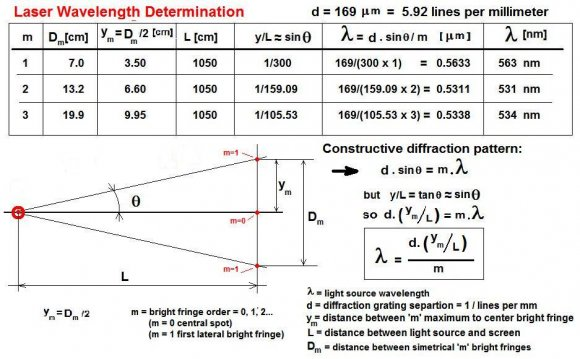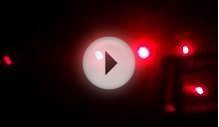# Laser diffraction grating experiment

February 19, 2023Light propagates, or travels, in waves. Waves have two main properties: frequency and wavelength. When you know one, it’s pretty easy to calculate the other.

When light waves overlap they create interference, and the patterns caused by this can be used to determine the wavelength of light. Conduct this cool diffraction grating experiment to determine the wavelength of laser light emitted from any laser pointer.

Calculate the wavelength of light.

• Diffraction grating with lines of known separation
• Laser pointer with a known wavelength
• Meter stick
• Ruler
• Binder clips
• Index card
• Tape
• Protractor
1. Tape an index card to the wall so the blank side is facing you.
2. Lay the meter stick on a table or the floor so the 0 meets the index card.
3. Mount the later pointer at the end of the meter stick, pointing towards the index card.
4. Mount the diffraction grating a few centimeters from the index card so the lines are vertical.
5. Turn off the lights in the room and turn on the laser pointer.
6. Use your protractor to measure the angle between the meter stick and the first order visible band.
7. Use the formula Where λ is the wavelength, in meters d is the distance in meters between lines on the diffraction grating is the angle and n is the order. Unless the room is extremely dark, you will only be able to see the first order, so n=1.
8. Compare your calculated wavelength to the wavelength provided by the manufacturer of the laser.
9. Repeat for different lengths along the meter stick.

Diffraction gratings diffract, or split, light periodically, meaning the light splits into several beams with a given angular separation. In this experiment, the first period, n=1, will be the brightest spot on the index card (besides the straight path of the laser, of course) after the grating splits the rays from the laser pointer. Using the formula above, you can verify the wavelength of light using what the manufacturer of the laser pointer says it is. If the room is dark enough, you may even be able to measure the 2nd and 3rd periods and plug n = 2 and n = 3 into your equation, respectively. It should yield the same result.

Source: www.education.com
##### RELATED VIDEODiffraction Grating demonstration - A level PhysicsDiffraction pattern of a He-Neon Laser due to a ...Lasers and Diffraction Gratings